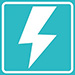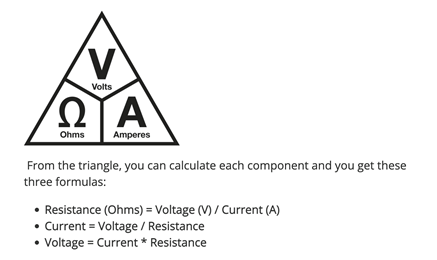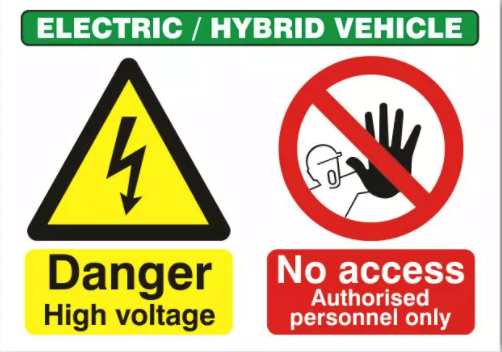##UNITS OF MEASUREMENT

###Ohms – An Ohm is the electrical unit used for measuring resistance, its symbol is Ω.

Watt – This is a unit of power and is denoted by use of the letter W.Voltage – Voltage is the difference in electrical potential between two different points, it can be thought of as electrical pressure, and it is denoted by the letter or U.

Amperes – This is the term used to describe the flow of electrical current, it is denoted by the letter A.

AC – Alternating Current is an electric current which reverses direction periodically. AC current is the way that electricity is distributed through the power network in the UK.  You will have this in your house and workplace.

DC – Direct Current is an electric current that travels in only one direction, this is the type of current that a battery produces.

##UNITS OF MEASUREMENT

###Ohms – An Ohm is the electrical unit used for measuring resistance, its symbol is Ω.

Watt – This is a unit of power and is denoted by use of the letter W.Voltage – Voltage is the difference in electrical potential between two different points, it can be thought of as electrical pressure, and it is denoted by the letter or U.

Amperes – This is the term used to describe the flow of electrical current, it is denoted by the letter A.

AC – Alternating Current is an electric current which reverses direction periodically. AC current is the way that electricity is distributed through the power network in the UK.  You will have this in your house and workplace.

DC – Direct Current is an electric current that travels in only one direction, this is the type of current that a battery produces.